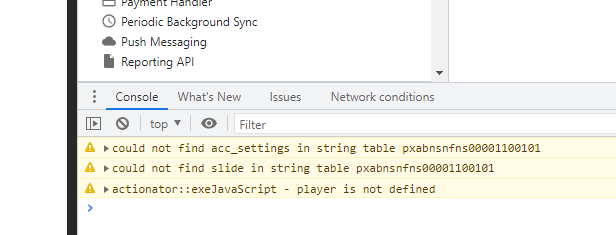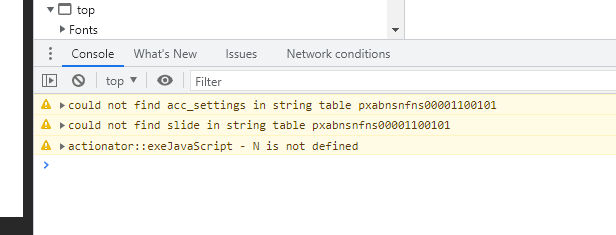# Exponential Operator for Adjusting Variable

I am trying to add a calculation that would calculate the loan amount based on various variables.  The equation is P * ((J) / (1-J)^n) .  There is not an exponential operator and I do not see at the moment how I would calculate this.  Has anyone experienced a similar issue and how did you solve it?

Any help is much appreciated.

###### 11 RepliesUsing a JavaScript trigger you should be able to calculate using your equation and push the results back into another variable.

Create variables for J, P, n, and output.

Create a JavaScript trigger to grab the values entered in for J, P, n and return the equation results in output.

var player=GetPlayer();

//grab the equation inputs from SL
var J=player.GetVar("J");
var P=player.GetVar("P");
var n=player.GetVar("n");
//run the calculation and push into the SL variable called output
results=P*(J/(Math.pow((1-J), n)));
player.SetVar("output",results);Hey Steve, thanks a lot for your help on this.  My final equation was:

//grab the equation inputs from SL
var P=player.GetVar("LoanAmount");
var J=player.GetVar("InterestRate");
var n=player.GetVar("NumberOfMonths")
//run the calculation and push into the SL variable called output
//results=(Math.pow((1+J),-n));
results=P*(J/(1-(Math.pow((1+J),-n))));
player.SetVar("MonthlyPayment",results);

This is a great function and will allow a ton of flexibility from the base functions.2 basic errors in your script you could have found yourself when using the browser console to test when publishing.

Publish for Web, open it up and open the Console. F12 or CTRL+Shift+IYou will notice the error... exeJavascript - player is not defined.
That points to an error in somewhere in your script you call the Storyline player, but donot declare it.

As Storyline triggers all have their own scope...you need to make sure you have access to the player in every trigger. Global scope can be achieved but thats for another time.

`var player = GetPlayer();`

After this change publishing again...you will notice it will not work.
And you have another error.
This one...In fact a similar error as before, but now indicating that the variable N is not defined.
Checking your code you immediately notice that there is a variable 'N'  but it is not called.
`var N=player.GetVar("N");`